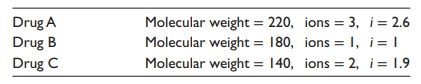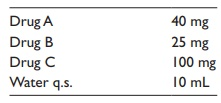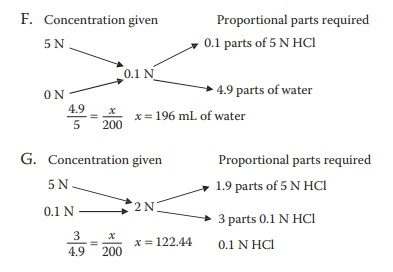| Home | | Pharmaceutical Drugs and Dosage | | Pharmaceutical Industrial Management |

## Chapter: Pharmaceutical Drugs and Dosage: Pharmacy math and statistics

Pharmaceutical Drugs and Dosage: Pharmacy math and statistics - Review questions and answers

Review questions

5.1 Amoxicillin suspension.

A. How much water would need to be added to a bottle contain-ing 12.5 g of dry powder for reconstitution into a 250 mg/5 mL suspension?

Hint: Use ratio and proportion, and remember to use the same units.

B. How many milliliters of amoxicillin suspension containing 250 mg/5 mL must be administered to a patient in need of a 400-mg dose of amoxicillin?

Hint: Use ratio and proportion.

C. If each 5 mL of a 250 mg/5 mL reconstituted amoxicillin suspen-sion contains 0.15 mEq of sodium, how much sodium does it rep-resent in mg?

Hint: Use the atomic weight of sodium.

D. Given your answers to (a) and (b) above, how much sodium would the patient be taking per day if the patient is dosed 400 mg t.i.d.? Hint: Use ratio and proportion.

5.2 Cyclophosphamide tablets.

A. Cyclophosphamide is available as 50-mg tablets and has a recom-mended dose of 5 mg/kg o.d. What would be the daily dose for a 175-lb patient?

Hint: Use proportion after converting every quantity to same units.

B. How many tablets should be dispensed for a dosage regimen of 10 days?

Hint: Calculate the number of tablets per day first.

5.3 Dosage for Children.

For a drug with the adult dose of 100 mg/kg, what would be the dose for a 4-feet-tall 8-year-old child weighing 80 lbs? Calculate using the nomogram, Fried’s rule, Young’s rule, and Clark’s rule.

A. Calculate the NaCl equivalents (E value) for the following three drugs, given that NaCl has a molecular weight of 58.5 and dis-sociates into two ions, with a dissociation constant (i) of 1.8.B. For the prescription noted below, calculate the NaCl equivalents present in the formulation.C. Calculate the amount of NaCl equivalents that would need to be added to the above formulation to make it isotonic for ophthalmic administration.

D. If NaCl were incompatible with one or more of drugs, how much dextrose (molecular weight = 180) may be used instead.

5.5 Volume and weight interconversions

A. Glycerin is a highly viscous liquid that may be weighed instead of measured in volume. How much of weight of glycerin would be equivalent to 4.6 mL of its volume, given that the density of glycerin at room temperature is 1.26 g/cm3?

B. Ethanol is a low-viscosity liquid that is easier measured in vol-ume than in weight. Given that its density is 0.78 g/cc, how much volume of ethanol is needed to prepare 25 mL of a 5% v/v solution?

C. Ethanol is a low-viscosity liquid that is easier measured in volume than in weight. Given that its density is 0.78 g/cc, how much vol-ume of ethanol is needed to prepare 25 g of a 5% w/w solution?

5.6 Concentration calculations

A. What would be the equivalent weight of calcium chloride (CaCl2) if its molecular weight is 111 g/mol?

B. What amount of CaCl2 would be needed to make 50 mL of a 0.5 M solution?

C. What amount of CaCl2 would be needed to make 50 mL of a 0.5 N solution?

D. A drug product was found to contain 40 ppm of an impurity dur-ing analysis. How many milligrams of this impurity might be ingested by an average 150-lb adult human being if the drug is to be administered in doses of 5 mg/kg/day in four divided doses?

E. What is the mole fraction of an isotonic NaCl solution? Molecular weight of NaCl is 58.5 and that of water is 18.

Hint: Isotonic NaCl solution has 0.9% w/v salt concentration.

F. How much purified water is needed to prepare 200 mL of 0.1 N HCl solution from its 5 N stock solution?

G. How much of the 0.1 N HCl solution would be needed to prepare 200 mL of a 2 N solution, using the 5 N stock solution of HCl?

5.7 Calculate the mean, median, variance, and standard deviation of fol-lowing sets of values:

A. 2.6, 4.2, 3.7, 1.7, 3.2

B. 12.8, 9.6, 15.7, 14.8, 13.2

C. 3.2, 4.9, 12.4, 16.8, 9.3

D. By reviewing above results, which of the three data sets has the highest spread around the central tendency?

E. By reviewing the above results, which of the three data sets has the least spread around the central tendency?

F. By reviewing the above results, the differences between the means of which two data sets are most likely to be statistically significant?

G. By reviewing the above results, the differences between the means of which two data sets are least likely to be statistically significant?

5.1 A. 12.5 g × 1,000 mg/1 g = 12,500 mg × mL/50 mg = 250 mL

B. 400 mg × 5 mL/250 mg = 8 mL

C. 0.15 × 23 = 3.45 mg

D. 250/0.15 = 1200/x, x = 4.8 mEq of sodium = 4.8 × 23 = 110.4 mg

5.2 A. 5 mg/kg× 175 lb × 1 kg/2.20 lb = 398 mg daily dose

B. Number of tablets per day = 398/50 = 8 tablets, Tablets for 10 days = 8 × 10 = 80 tablets

5.3 Child’s dose = Adult dose × Child’s BSA in m2 /1.73m25.4 Sodium chloride equivalent = Molecular weight of sodium chloride × i factor of drug/i factor of sodium chloride × molecular weight of drug

a. E value = 58.5 × 1.8/220 × 2.6 = 0.18

E value = 58.5 × 1.8/180 × 1 = 0.58

E value = 58.5 × 1.8/140 × 1.9 = 0.40

B. Sodium chloride equivalent = (40 × 0.18 + 25 × 0.58 + 100 × 0.40) × 10 − 3 = 0.0617 sodium chloride equivalent

C. Amount of NaCl equivalent = (0.09 – 0.0617) = 0.0283 g

D. E value of dextrose = 58.5 × 1.8/180 × 1.9 = 0.31

1/0.31 = (x/0.0283)  x = 0.091g of dextrose

5.5 A. 4.6 mL × 1.26 g/cm3 = 5.796 g

B. 25 mL × 5 g/100 mL = 1.25 g

1.25g/0.78 = 1.60 mL

C. 25 mL × 5/100 = 1.25 g

1.25 g/0.78 = 1.60 mL

5.6 A. 111/2 = 55.5 g

B. 2.775 g

C. 1.3875 g

D. Total dose = 5 mg/kg/day × 150 lb × 0.455 kg/lb = 340 mg/day

Impurity = 340 mg/day × 40 mg/kg = 0.0136 mg/day

E. Mole fraction = Moles of NaCl/(Moles of NaCl + Moles of water) = 0.015/(0.015 + 5.50) = 0.00275.7 A. i. Mean = 3.08

Median = 3.2

Variance = 0.95

Standard deviation = 0.97

ii. Mean = 13.22

Median = 13.2

Variance = 5.48

Standard deviation = 2.34

iii. Mean = 9.32

Median = 9.3

Variance = 30.60

Standard deviation = 5.53

B. Dataset c has the highest spread around the central tendency.

C. Dataset a has the least spread around the central tendency.

D. Differences between the means of datasets a and b are most likely to be statistically significant.

E. Differences between the means of datasets b and c are least likely to be statistically significant.